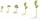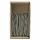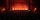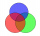# Calculator expression with brackets

The calculator performs basic and advanced operations with fractions, expressions with fractions combined with integers, decimals, and mixed numbers. It also shows detailed step-by-step information about the fraction calculation procedure. Solve problems with two, three, or more fractions and numbers in one expression.

### 1/3 * (1/2 - 33/8) = -23/24 ≅ -0.9583333

Spelled result in words is minus twenty-three twenty-fourths.

### How do you solve fractions step by step?

1. Conversion a mixed number 3 3/8 to a improper fraction: 3 3/8 = 3 3/8 = 3 · 8 + 3/8 = 24 + 3/8 = 27/8

To find a new numerator:
a) Multiply the whole number 3 by the denominator 8. Whole number 3 equally 3 * 8/8 = 24/8
b) Add the answer from previous step 24 to the numerator 3. New numerator is 24 + 3 = 27
c) Write a previous answer (new numerator 27) over the denominator 8.

Three and three eighths is twenty-seven eighths
2. Subtract: 1/2 - 27/8 = 1 · 4/2 · 4 - 27/8 = 4/8 - 27/8 = 4 - 27/8 = -23/8
For adding, subtracting, and comparing fractions, it is suitable to adjust both fractions to a common (equal, identical) denominator. The common denominator you can calculate as the least common multiple of both denominators - LCM(2, 8) = 8. In practice, it is enough to find the common denominator (not necessarily the lowest) by multiplying the denominators: 2 × 8 = 16. In the following intermediate step, it cannot further simplify the fraction result by canceling.
In other words - one half minus twenty-seven eighths = minus twenty-three eighths.
3. Multiple: 1/3 * the result of step No. 2 = 1/3 * (-23/8) = 1 · (-23)/3 · 8 = -23/24
Multiply both numerators and denominators. Result fraction keep to lowest possible denominator GCD(-23, 24) = 1. In the following intermediate step, it cannot further simplify the fraction result by canceling.
In other words - one third multiplied by minus twenty-three eighths = minus twenty-three twenty-fourths.

#### Rules for expressions with fractions:

Fractions - simply use a forward slash between the numerator and denominator, i.e., for five-hundredths, enter 5/100. If you are using mixed numbers, be sure to leave a single space between the whole and fraction part.
The slash separates the numerator (number above a fraction line) and denominator (number below).

Mixed numerals (mixed fractions or mixed numbers) write as integer separated by one space and fraction i.e., 1 2/3 (having the same sign). An example of a negative mixed fraction: -5 1/2.
Because slash is both signs for fraction line and division, we recommended use colon (:) as the operator of division fractions i.e., 1/2 : 3.

Decimals (decimal numbers) enter with a decimal point . and they are automatically converted to fractions - i.e. 1.45.

The colon : and slash / is the symbol of division. Can be used to divide mixed numbers 1 2/3 : 4 3/8 or can be used for write complex fractions i.e. 1/2 : 1/3.
An asterisk * or × is the symbol for multiplication.
Plus + is addition, minus sign - is subtraction and ()[] is mathematical parentheses.
The exponentiation/power symbol is ^ - for example: (7/8-4/5)^2 = (7/8-4/5)2

The calculator follows well-known rules for order of operations. The most common mnemonics for remembering this order of operations are:
PEMDAS - Parentheses, Exponents, Multiplication, Division, Addition, Subtraction.
BEDMAS - Brackets, Exponents, Division, Multiplication, Addition, Subtraction
BODMAS - Brackets, Of or Order, Division, Multiplication, Addition, Subtraction.
GEMDAS - Grouping Symbols - brackets (){}, Exponents, Multiplication, Division, Addition, Subtraction.
Be careful, always do multiplication and division before addition and subtraction. Some operators (+ and -) and (* and /) has the same priority and then must evaluate from left to right.

## Fractions in word problems:

• Farmer PeterFarmer Peter paints 12 chicken coop. He started painting this day morning. Now he only has 1/4 of the chicken coop left to paint this afternoon. How many chicken coops did farmer Peter paint this morning?
• SeedsOf the 72 wet seeds, 7/8 germinated. How many seeds germinate?
• Tablespoon of saltMrs. Monika uses 3/4 tablespoons of salt for every kilogram of ground pork. How many tablespoons of salt will she use for 3 1/2 kilograms of ground pork?
• Evaluate expressionEvaluate expression using BODMAS rule: 1 1/4+1 1/5÷3/5-5/8
• NailsIf a keg of nails costs \$129.45 and 6/7 of the keg is used, what is the value of the remaining nails?
• The frameRodney has a board that is 5/6 yards long. He cuts 1/5 yard off the board and uses the rest of the board to make a frame. How much of the board is used to make the frame?
• A large 2A large popcorn bag holds four times as much as a small popcorn bag at the end of the party 3 1/3 small bags and 2 1/4 large bags left. How many small bags with the leftover popcorn fill?
• Complicated sum minus productWhat must be subtracted from the sum of 3/8 and 5/16 to get difference equal to the product of 5/8 and 3/16?
• Evaluate 17Evaluate 2x+6y when x=- 4/5 and y=1/3. Write your answer as a fraction or mixed number in simplest form.
• There 11There are 50 pupil in the class. Out of of this number,1/10 speak French only and 4/5 of the 10 remainder speak both French and English . If the rest speak English only find the number of students who speak only English?
• The cost 2The cost of 5 apples is \$3.45, and 5 oranges are \$1.23. If Rachel buys one apple and one orange, then how much must she pay?
• One third 2One third of all students in class live in a house. If here are 42 students in a class, how many of them live in house?
• Fraction expressionWhich expression is equivalent to : minus 9 minus left parenthesis minus 4 start fraction 1 divided by 3 end fraction right parenthesis